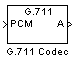G711 Codec

Quantize narrowband speech input signals

Library

Quantizers

dspquant2

•Description

The G711 Codec block is a logarithmic scalar quantizer designed for narrowband speech. Narrowband speech is defined as a voice signal with an analog bandwidth of 4 kHz and a Nyquist sampling frequency of 8 kHz. The block quantizes a narrowband speech input signal so that it can be transmitted using only 8-bits. The G711 Codec block has three modes of operation: encoding, decoding, and conversion. You can choose the block's mode of operation by setting the Mode parameter.

If, for the Mode parameter, you choose Encode PCM to A-law, the block assumes that the linear PCM input signal has a dynamic range of 13 bits. Because the block always operates in saturation mode, it assigns any input value above ${2}^{12}-1$ to ${2}^{12}-1$ and any input value below $-{2}^{12}$ to $-{2}^{12}$. The block implements an A-law quantizer on the input signal and outputs A-law index values. When you choose Encode PCM to mu-law, the block assumes that the linear PCM input signal has a dynamic range of 14 bits. Because the block always operates in saturation mode, it assigns any input value above ${2}^{13}-1$ to ${2}^{13}-1$ and any input value below $-{2}^{13}$ to $-{2}^{13}$. The block implements a mu-law quantizer on the input signal and outputs mu-law index values.

If, for the Mode parameter, you choose Decode A-law to PCM, the block decodes the input A-law index values into quantized output values using an A-law lookup table. When you choose Decode mu-law to PCM, the block decodes the input mu-law index values into quantized output values using a mu-law lookup table.

If, for the Mode parameter, you choose Convert A-law to mu-law, the block converts the input A-law index values to mu-law index values. When you choose Convert mu-law to A-law, the block converts the input mu-law index values to A-law index values.

Note

Set the Mode parameter to Convert A-law to mu-law or Convert mu-law to A-law only when the input to the block is A-law or mu-law index values.

If, for the Mode parameter, you choose Encode PCM to A-law or Encode PCM to mu-law, the Overflow diagnostic parameter appears on the block parameters dialog box. Use this parameter to determine the behavior of the block when overflow occurs. The following options are available:

• Ignore — Proceed with the computation and do not issue a warning message.

• Warning — Display a warning message in the MATLAB® Command Window, and continue the simulation.

• Error — Display an error dialog box and terminate the simulation.

Note

Like all diagnostic parameters on the Configuration Parameters dialog box, Overflow diagnostic parameter is set to Ignore in the code generated for this block by Simulink® Coder™ code generation software.

Parameters

Mode
• When you choose Encode PCM to A-law, the block implements an A-law encoder.

• When you choose Encode PCM to mu-law, the block implements a mu-law encoder.

• When you choose Decode A-law to PCM, the block decodes the input index values into quantized output values using an A-law lookup table.

• When you choose Decode mu-law to PCM, the block decodes the input index values into quantized output values using a mu-law lookup table.

• When you choose Convert A-law to mu-law, the block converts the input A-law index values to mu-law index values.

• When you choose Convert mu-law to A-law, the block converts the input mu-law index values to A-law index values.

Overflow diagnostic

Use this parameter to determine the behavior of the block when overflow occurs.

• Select Ignore to proceed with the computation without a warning message.

• Select Warning to display a warning message in the MATLAB Command Window and continue the simulation.

• Select Error to display an error dialog box and terminate the simulation.

This parameter is only visible if, for the Mode parameter, you select Encode PCM to A-law or Encode PCM to mu-law.

References

ITU-T Recommendation G.711, “Pulse Code Modulation (PCM) of Voice Frequencies,” General Aspects of Digital Transmission Systems; Terminal Equipments, International Telecommunication Union (ITU), 1993.

Supported Data Types

PortSupported Data Types

PCM

• 16-bit signed integers

A

• 8-bit unsigned integers

mu

• 8-bit unsigned integers

 Quantizer (Simulink) Simulink Scalar Quantizer Decoder DSP System Toolbox Uniform Decoder DSP System Toolbox Uniform Encoder DSP System Toolbox Vector Quantizer Decoder DSP System Toolbox Vector Quantizer Encoder DSP System Toolbox

Extended Capabilities

C/C++ Code GenerationGenerate C and C++ code using Simulink® Coder™.

Introduced before R2006a

DSP System Toolbox DocumentationGet trial now